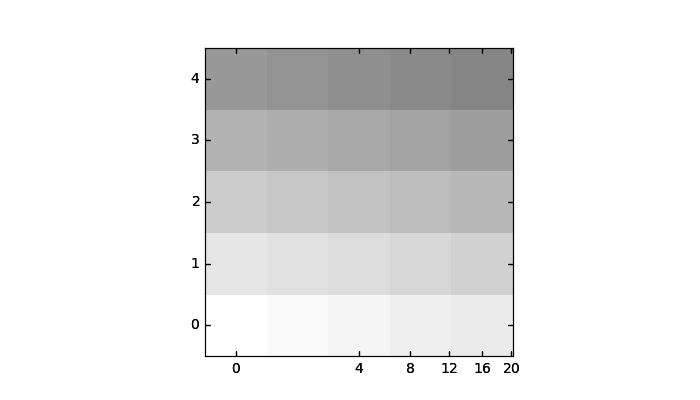Travis-CI:# Demo Curvelinear Grid2¶

Custom grid and ticklines.

This example demonstrates how to use GridHelperCurveLinear to define custom grids and ticklines by applying a transformation on the grid. As showcase on the plot, a 5x5 matrix is displayed on the axes.```import numpy as np
import matplotlib.pyplot as plt

from mpl_toolkits.axisartist.grid_helper_curvelinear import \
GridHelperCurveLinear
from mpl_toolkits.axisartist.axislines import Subplot

import mpl_toolkits.axisartist.angle_helper as angle_helper

def curvelinear_test1(fig):
"""
grid for custom transform.
"""

def tr(x, y):
sgn = np.sign(x)
x, y = np.abs(np.asarray(x)), np.asarray(y)
return sgn*x**.5, y

def inv_tr(x, y):
sgn = np.sign(x)
x, y = np.asarray(x), np.asarray(y)
return sgn*x**2, y

extreme_finder = angle_helper.ExtremeFinderCycle(20, 20,
lon_cycle=None,
lat_cycle=None,
# (0, np.inf),
lon_minmax=None,
lat_minmax=None,
)

grid_helper = GridHelperCurveLinear((tr, inv_tr),
extreme_finder=extreme_finder)

ax1 = Subplot(fig, 111, grid_helper=grid_helper)
# ax1 will have a ticks and gridlines defined by the given
# transform (+ transData of the Axes). Note that the transform of
# the Axes itself (i.e., transData) is not affected by the given
# transform.

ax1.imshow(np.arange(25).reshape(5, 5),
vmax=50, cmap=plt.cm.gray_r,
interpolation="nearest",
origin="lower")

# tick density
grid_helper.grid_finder.grid_locator1._nbins = 6
grid_helper.grid_finder.grid_locator2._nbins = 6

if 1:
fig = plt.figure(1, figsize=(7, 4))
fig.clf()

curvelinear_test1(fig)
plt.show()
```

Total running time of the script: ( 0 minutes 0.093 seconds)

Gallery generated by Sphinx-Gallery